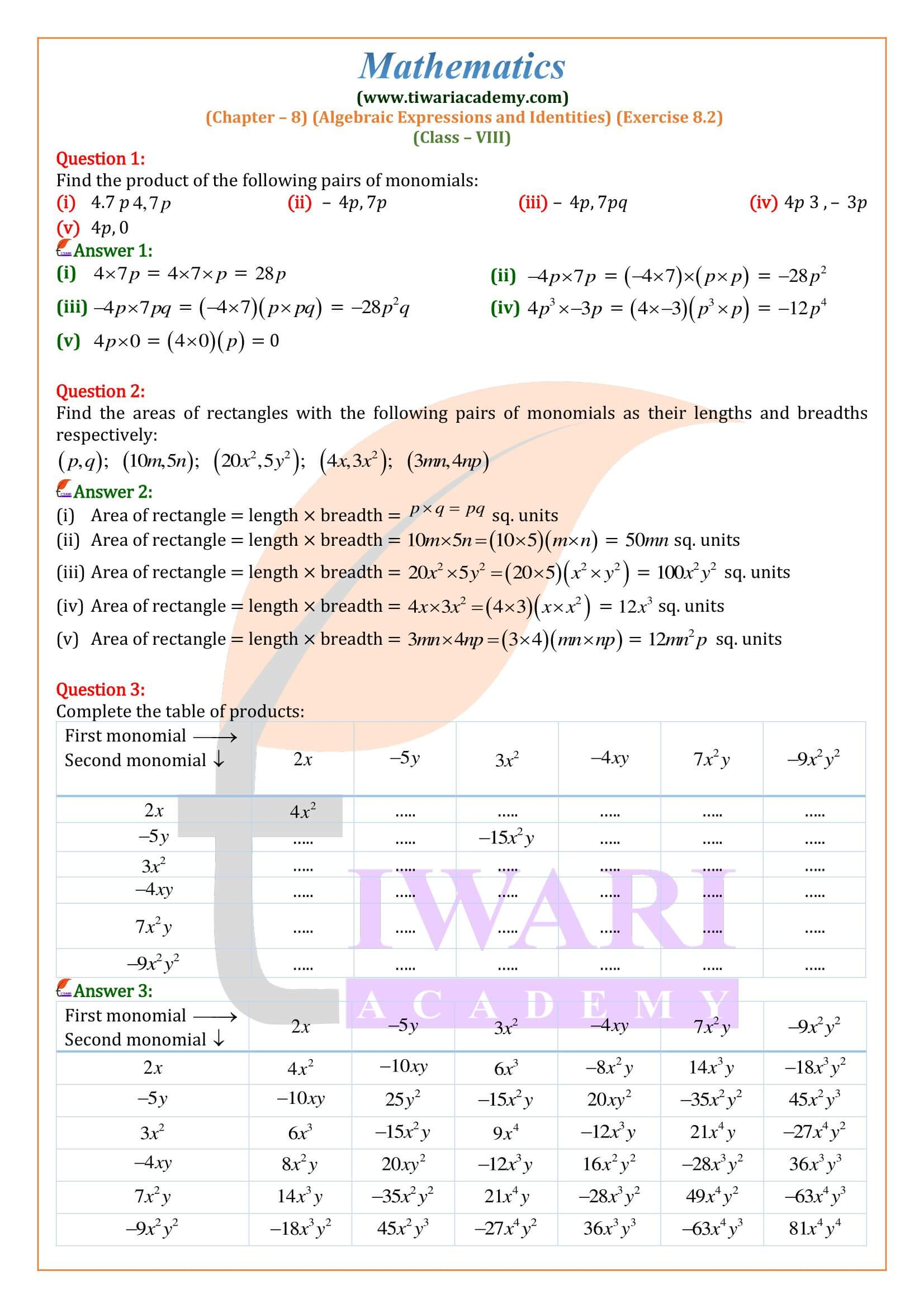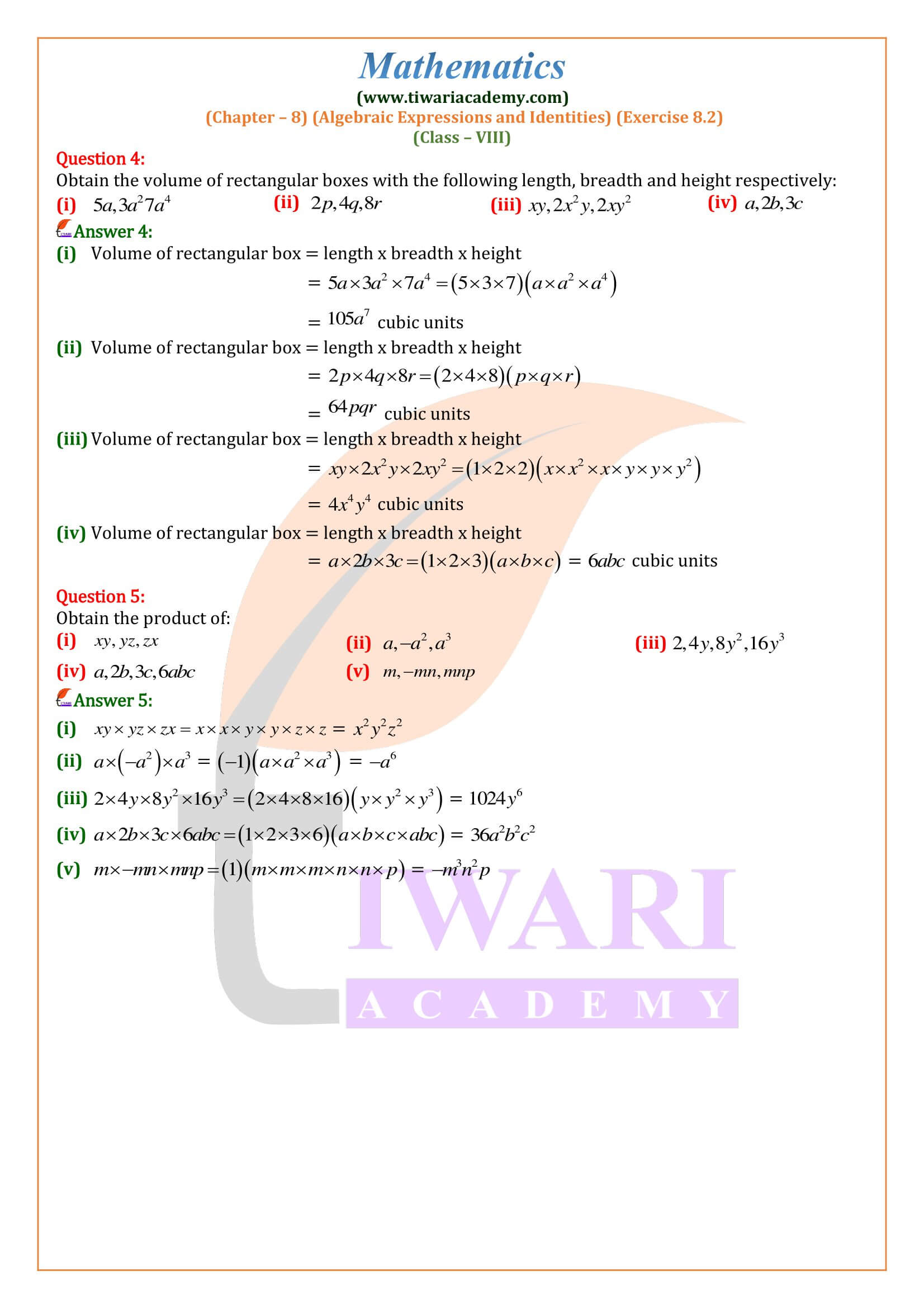CBSE NCERT Solutions for Class 8 Maths Chapter 8 Exercise Algebraic Expressions and Identities 8.2 in Hindi and English Medium updated for session 2023-24. Class 8 Maths ex. 8.2 solution is revised on the basis of new syllabus and latest NCERT issued for CBSE 2023-24.

• ## 8th Maths Exercise 8.2 Solution in Hindi and English Medium

 Class: 8 Mathematics Chapter: 8 Exercise: 8.2 Topic Name: Algebraic Expressions and Identities Content: PDF and Video Solutions Academic Session: CBSE 2023-24 Medium: Hindi and English Medium

### Class 8 Maths Chapter 8 Exercise 8.2 Solution

Class VIII Mathematics textbook Ex. 8.2 Algebraic Expressions and Identities in PDF format free to download without any login. Videos related to all exercises are also given with text solutions explaining each question properly. All the questions of Exercise 8.2 class 8 Maths are done as per CBSE guideline. Step by step calculation is shown for better understanding. These solutions are also helpful for state board students.

#### Degree of a Polynomial

The highest power of variable of a polynomial in one variable and the highest power of the sum of the exponents of the variables of a polynomial in more than one variable is called the degree of the polynomial.
Examples:

1. Degree of 6×7 is 7. 2 3
2. Degree of – 3×2 y3 is 2 + 3 = 5.
3. Degree of the polynomial 4xy2 – 5×2 y2 + 3×3 y – 7 is 4.
##### To find the Value of an algebraic expression

The algebraic expressions have variables and constant terms. To find the numerical value of an algebraic expression, the values of variables should be known.

###### To find the value of an algebraic expression the following steps are required:

(i) Replace the variable with its value.
(ii) Solve each term.
(iii) Simplify the arithmetic expression.

e.g., If x = 2, y = – 1, to find the numerical value of the expression x² y – xy, we substitute the values of x and y thus, x² y – xy = (2)² (–1) – (2) (–1) = – 4 + 2 = – 2.

While adding algebraic expressions we collect the like terms and add them. The sum of several like terms is the like term whose coefficient is the sum of the coefficients of these like terms.

###### Subtraction of Algebraic Expressions

Steps:

• (i) Arrange the terms of the given expressions in the same order.
• (ii) Write the given expressions in two rows in such a way that the like terms occur one below the other, keeping the expression to be subtracted in the second row.
• (iii) Change the sign of each term in the lower row from + to – and from – to +.
• (iv) With new signs of the terms of lower row, add column
wise.

### What do you learn about algebraic subtraction in exercise 8.2?

In class 8 Maths exercise 8.2 algebraic subtraction, we subtract one algebraic expression from the other by comparing the like terms.
For example:
Subtract 4a + 5b 3c from 6a 3b + c.
This can be solved in the following manner:
We have: 6a – 3b + c and 4a + 5b – 3c
6a – 3b + c – (+ 4a + 5b – 3c) = 2a – 8b + 4c

### Is exercise 8.2 of 8th Maths explains about the degree of a polynomial?

The exercise 8.2 of class 8 mathematics explains briefly about the degree of polynomial. It explains that in the case of a polynomial with more than one variable, the degree is found by looking at each monomial within the polynomial, adding together all the exponents within a monomial, and choosing the largest sum of exponents. That sum is the degree of the polynomial.

### Can we find the degree of linear equation using the tricks of exercise 8.2 of class 8 Maths?

In Algebra, the degree is the largest exponent of the variable in the given equation. … If the equation has a degree of one, it is a linear equation. In short, the degree of linear equations is always one. For example, 3x + 10 = z, has a degree 1 so it is a linear equation.Last Edited: April 17, 2023